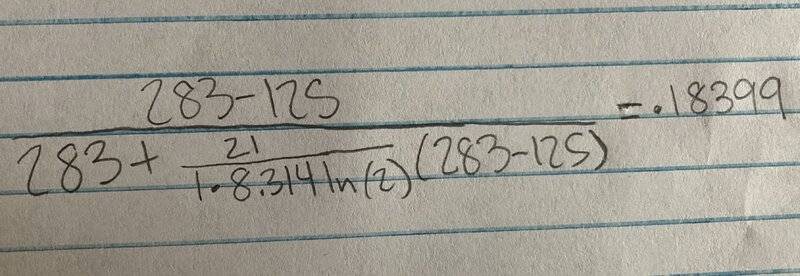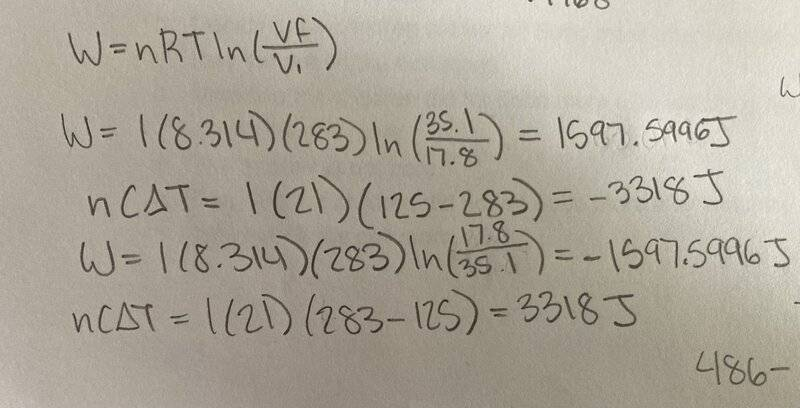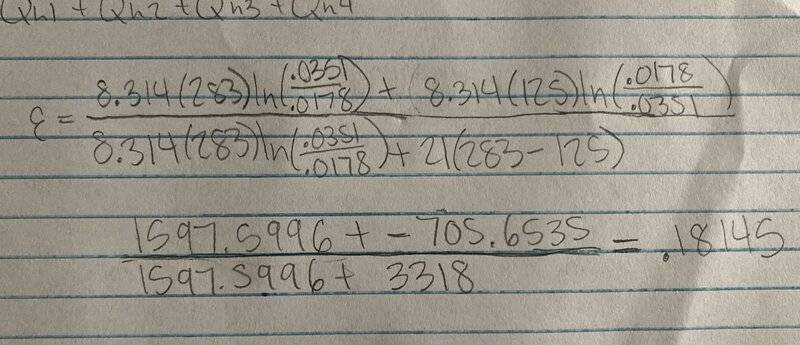# Finding efficiency of an engine (with attempt)

• RandiSS

#### RandiSS

Homework Statement
An engine using 1 mol of an ideal gas ini- tially at 17.8 L and 283 K performs a cycle consisting of four steps:
1) an isothermal expansion at 283 K from 17.8 L to 35.1 L;
2) cooling at constant volume to 125 K ;
3) an isothermal compression to its original volume of 17.8 L; and
4) heating at constant volume to its original temperature of 283 K .
Find its efficiency. Assume that the heat capacity is 21 J/K and the univer- sal gas constant is 0.08206 L · atm/mol/K = 8.314 J/mol/K.
Relevant Equations
ɛ=W/Q
W=nRTln(Vf/Vi)
Q=nCΔTThis was my first attempt which was not right. But it was at least a percent unlike my 2nd attempt.This was my second which I found on another forum website. It said it found the work of step 1, heat absorbed of step 2, work of step 3, and absorbed heat of step 4. However, the answers to the equations they provided just canceled out when finding the total heat absorbed and work done, which gave me an undefined efficiency. I know I need to find the work and heat for each step, combine, then divide total W by total Q. Am I using the wrong numbers or is this just not the right process to find efficiency?

Update:I think I might have figured it out but I’m not sure.

Update:View attachment 324922
I think I might have figured it out but I’m not sure.
I got it!

•berkeman
where you get 0.0315 and 0.0178 from?

where you get 0.0315 and 0.0178 from?
"an isothermal expansion at 283 K from 17.8 L to 35.1 L"
For some reason, @RandiSS converted to kilolitres, but since only the ratio matters it makes no difference.

•berkeman
where you get 0.0315 and 0.0178 from?
Maybe from here?
1) an isothermal expansion at 283 K from 17.8 L to 35.1 L;